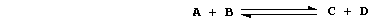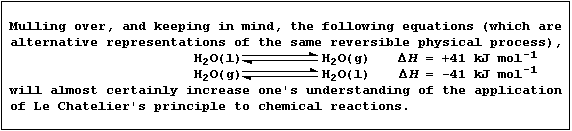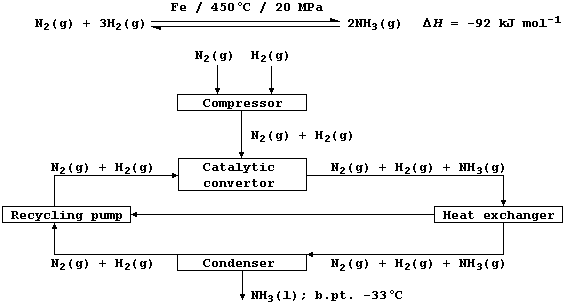```SELECTED PRINCIPLES: EQUILIBRIA (1)
A chemical reaction can be executed in three types of system. First,
an isolated system, which is one that does not allow either energy
or matter to be transferred (to its surroundings); an example, albeit
imperfect, is a thermos flask. Second, a closed system, which is one
that allows energy but not matter to be transferred; e.g., a sealed
conical flask. And third, an open system, which is one that allows both
energy and matter to be transferred; e.g., an open conical flask.
In principle, each chemical reaction is reversible; i.e., it can occur
in both directions. Consider this abstract reaction in a closed system:``````When the two reactants A and B are mixed, only the forward reaction is
possible. As it continues, the numbers of A and B molecules decrease:
so, the forward reaction slows down. At the same time, the numbers of
C and D molecules increase: so, the reverse reaction speeds up. And,
at a constant temperature and pressure, the system eventually reaches a
state of dynamic equilibrium; i.e., the forward and reverse reactions
occur at equal rates, and the net concentrations of A, B, C, and D
molecules remain constant.
The term 'position of equilibrium' refers to one particular state of
a closed system; and one state, or position, differs from another in
the concentrations of substances present in the equilibrium mixture.
Concentration, pressure, and temperature are three of the independent
variables known to affect the position of equilibrium; the qualitative
effect of changing such variables is summarized by Le Chatelier's
Principle: 'When a closed system at dynamic equilibrium is subjected to
change in an independent variable, the position of equilibrium shifts
so as to oppose the effect of the imposed change.'.
Thus, at dynamic equilibrium in a closed system,
... an increase in the concentrations of products shifts the position
of equilibrium to the left.
... an increase in the concentrations of reactants shifts the position
of equilibrium to the right.
... an increase in pressure increases the yield when there are fewer
moles of gas on the product side of the equation.
... an increase in pressure decreases the yield when there are more
moles of gas on the product side of the equation.
... a change in pressure has no effect on the yield when there are the
same number of moles of gas on each side of the equation.
... an increase in temperature increases the yield when the reaction is
endothermic (+ DH)
... an increase in temperature decreases the yield when the reaction is
exothermic (- DH).``````1.  Shown below is a flow diagram for the manufacture of ammonia by the
Haber process; and the following equation summarizes its synthesis by
the set of reaction conditions most commonly used in industry.``````(a) Dinitrogen (b.pt. -196°C) is obtained by fractional distillation of
liquid air. State two methods of obtaining dihydrogen (b.pt. -253°C).
_______________________________________________________________________

(b) Using the conditions summarized in the above equation, the yield of
ammonia is about 28% at equilibrium. Complete the following sentences,
so as to summarize the effects of three independent variables, on the
yield of ammonia and the rate of reaction, in this process.
The high pressure used __________ the yield, because the position of
equilibrium moves to oppose the increase in pressure, and __________
the rate, because there are more collisions between the particles.
The high temperature used __________ the yield, because the position of
equilibrium moves to oppose the increase in temperature of this
exothermic reaction, but __________ the rate, because more particles
have the required activation energy for successful collisions.
The catalyst used has __________ on the yield, because it affects the
forward and reverse reactions equally, but __________ the rate, because
it lowers the activation energy and so more particles have the required
energy for successful collisions.

2.  A research chemist prepared a sample of labelled ammonia, using the
isotope nitrogen-15. This sample was heated with iron, at 450°C under
high pressure (20 MPa) in a closed system, until the composition of the
mixture was constant. For the reaction studied, construct the symbol
equation complete with heat energy change. ____________________________
_______________________________________________________________________
_______________________________________________________________________

3.  Ammonia, a colourless gas, is very soluble in water (approximately
68000 cmł dissolves in 100 cmł of water at 25°C and 100 kPa; cf. with
1.5 and 3.0 cmł for dinitrogen and dihydrogen, respectively). Ammonia's
exceptionally high solubility in water is attributable to 'hydrogen
bonding'; i.e., the weak electrostatic attraction between the nucleus
of a bonded hydrogen atom and a lone-pair of electrons of a bonded
non-hydrogen atom (typically, nitrogen, oxygen, or fluorine). Calculate
the concentration, in mol dm-ł, of a saturated solution of ammonia. ___
_______________________________________________________________________

Dr. R. Peters                   Next                     Contents' List```